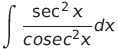Select Page

# CBSE Integrals Maths 12 Science Solutions for MCQ in English

CBSE Integrals Maths 12 Science Solutions for MCQ in English to enable students to get Solutions in a narrative video format for the specific question.

Expert Teacher provides CBSE Integrals Maths 12 Science Solutions for MCQ through Video Solutions in English language. This video solution will be useful for students to understand how to write an answer in exam in order to score more marks. This teacher uses a narrative style for a question from Integrals not only to explain the proper method of answering question, but deriving right answer too.

Please find the question below and view the Solution in a narrative video format.

Question:

Solution Video in English:

## Similar Questions from CBSE, 12th Science, Maths, Integrals

Question 1 :(View Answer Video)

Question 2 : Evaluate :(View Answer Video)

Question 3 : Write the value of:(View Answer Video)

Question 4 : Find :(View Answer Video)

Question 5 : Evaluate :(View Answer Video)

### Probability

Question 1 : A speaks truth in 75% of the cases, while B in 90% of the cases. In what percent of cases are they likely to contradict each other in stating the same fact? Do you think that statement of B is always true?     (View Answer Video)

Question 2 : One card is drawn from a pack of 52 cards. Find the probability of getting a king   (View Answer Video)

Question 3 : If P(F) = 0.35 and=0.85 and E and F are independent events. Find P(E).  (View Answer Video)

Question 4 : P speaks truth in 70% of the cases and Q in 80% of the cases. In what percent of cases are they likely to agree in stating the same fact?  (View Answer Video)

Question 5 : A bag A contains 4 black and 6 red balls and bag B 7 black and 3 red balls. A die is thrown. If 1 or 2 appears on it, then bag A is chosen, otherwise bag B. It two balls are drawn at random (without replacement) from the selected bag, find the probability of one of them being red and another black.        (View Answer Video)

### Continuity and Differentiability

Question 1 : Differentiate w.r.t.x the function. (View Answer Video)

Question 2 :  Find the second order derivative of the function. (View Answer Video)

Question 3 : Find the value of k, if the area of the triangle is 4 sq unit and vertices are (-2, 0), (0, 4), (0, k). (View Answer Video)

Question 4 :  Find the second order derivative of the function. (View Answer Video)

Question 5 : Ifand, find. (View Answer Video)

### Inverse Trigonometric Functions

Question 1 : Solve for(View Answer Video)

Question 2 : Ifthen find the value of x. (View Answer Video)

Question 3 : Iffind x. (View Answer Video)

Question 4 : Solve for(View Answer Video)

Question 5 : Ifthen write the value of x + y + xy. (View Answer Video)